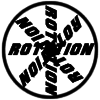#### You may also like### 8 Methods for Three by One

This problem in geometry has been solved in no less than EIGHT ways by a pair of students. How would you solve it? How many of their solutions can you follow? How are they the same or different? Which do you like best?### Rots and Refs

Follow hints using a little coordinate geometry, plane geometry and trig to see how matrices are used to work on transformations of the plane.### Reflect Again

Follow hints to investigate the matrix which gives a reflection of the plane in the line y=tanx. Show that the combination of two reflections in intersecting lines is a rotation.

# Nine Eigen

##### Age 16 to 18Challenge Level

A vector ${\bf v}$ is fixed by a matrix if

$$M{\bf v} = {\bf v}$$

The vector direction of ${\bf v}$ is fixed if there is some number $\lambda\neq 0$ such that

$$M{\bf v} = \lambda{\bf v}$$

This resource introduces concepts which appear in the later Further Pure Mathematics A Level modules.